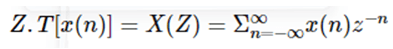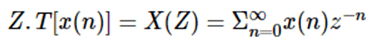Categories

# Matlab Z Transforms

Z transform is used to convert discrete time signals into the frequency domain. Discrete time signal includes real as well as imaginary values (Complex numbers). In Matlab ztrans function is used to find the z transform of any signal. We can pass one, two, or three arguments through ztrans function. Mathematically it performs just like Laplace transform, which is a discrete-time representation of Laplace transform’s transform is also used to plot poles and zeros which are important to represent regions of interest.

## Syntax:

• ztrans (sig)

`ztrans (input signal name)`

• ztrans (sig, x)

`ztrans (input signal name, transformation variable name)`

• ztrans (sig, var 1, x)

`ztrans (input signal name, variable name, transformation variable name)`

## How to Perform Z Transform in Matlab?

Z transform helps to perform analysis of linear time invariant systems or signals. it covers complex differential equations into simple mathematical equations. it can be unilateral or bilateral. If z transform is unilateral then it assumes a range between zero to infinity and if z transform is bilateral then the range is between minus infinity to plus infinity.……… (1)……… (2)

The above equations are used to calculate the z transform of LTI systems, equation 1 is applicable for bilateral systems, and equation two is applicable for unilateral systems. To calculate z transform we can use any type of input equation or signal, vectors, arrays, multi-dimensional matrices are also allowed as input functions. by using the above equations we can convert the discrete domain into the frequency domain similarly we can also convert the frequency domain signal into a discrete-time domain signal by using ‘iztrans’ function.

## Examples of Matlab Z Transform

Following are the examples are given below:

## Example 1:

In this example we have assumed a simple input signal which is a cosine function. Input function declared as ‘sig’ variable. To create a discrete-time function we need one symbolic variable .therefore we created one symbolic variable ‘n’ so the input signal becomes cosine of ‘n’. Then we applied ztrans function on the input signal. We get the mathematical equation in form of z. the output of ztrans function is in the frequency domain, it gives numerator as well as denominator values.

## Code:

``````clc ;
clear all ;
close all ;
syms n
sig = cos (n);
disp('z transform')
ztrans(sig)``````

## Example 2:

In example 2, two symbolic variables are declared var1 and var2. Variables var 1 and var 2 are used to create input functions. Here input function is an exponential function that is represented by exp syntax. Both the variables passed through exp function. The input function is declared by the variable ‘sig’. z transform of an exponential function is also represented in form of z.

## Code:

``````clc ;
clear all ;
close all ;
syms var1 var2
sig = exp(var1+var2);
disp('z transform')
ztrans(sig)``````

## Example 3:

In this example also two symbolic variables are declared var1 and var2. Variables var 1 and var 2 are used to create input functions. Here input function is exponential function which is represented by exp syntax. Both the variables passed through exp function. input function is declared by variable ‘sig’. two arguments are passed through the function input signal and one independent variable. In the previous example z transform was in form of z only but in this example, z transform is in form of independent variable ‘x’ and the rest algebraic equation is the same.

## Code:

``````clc ;
clear all ;
close all ;
syms var1 var2 x
sig = exp (var 1 + var 2);
disp('z transform')
ztrans(sig, x)``````

## Example 4:

In this example, three symbolic variables are used, which are var 1, var2, and x. Variables var 1 and var 2 are used to create the input function. Here input function is an exponential function that is represented by exp syntax. Both the variables and one independent variable passed through ‘exp’ function. The difference between the previous example and this example is arguments passed through function. In the previous example, only two variables passed through the function, and in this example, all the symbolic variables passed through ‘z trans’ function. Therefore it gives different algebraic equations as z transform. As the algebraic equation is different, we will different poles and zeros location and region of interest. The above example is illustrated below.

## Code:

``````clc ;
clear all ;
close all ;
syms var1 var2 x
sig = exp(var1 + var2 ) ;
disp( 'z transform')
ztrans (sig, var 1, x)``````